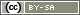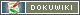# Score Responses

In most socio-scientific surveys, we are interested in the participant's opinion. This is not the case if you want to provide feedback on a knowledge test. These have correct and incorrect answers, and a score can be determined. In live analyses (e.g. Thurstone or Likert scales), sometimes a score can also be kept. An analysis of the personality test at the end of the questionnaire can create an incentive.

Tip The PHP code examples on this page look extremely lengthy – this is because each step is described separately with comments. Do not be alarmed by the length.

Tip Read the chapters Introduction to PHP Code and Introduction to Arrays to understand the code examples used.

Tip: If you just want to calculate the scale indices for an analysis, the functions `valueSum()` and `valueMean()` can be used to do so.

The simplest way of keeping score is for a quiz or a knowledge test. These have a range of selection questions and one of the options is correct for each one.

The following PHP code initially defines which questions will be evaluated and what the correct response is. An associative array is used accordingly. The arrays are defined here with square brackets (`[]`), the notation with `array()` would also be possible. The variable ID (for selection questions this is the question ID) is used as the key for each entry in the array. Each answer code that will be counted as “correct” is assigned as the value.

Following this, all questions are checked in a FOR loop – this is significantly more compact than having a dozen IF constructions.

```// Define questions and the correct answers
\$questions = [
'AB01' => 3,  // correct answer to question AB01 is 3
'AB02' => 1,  // correct answer to AB02 is code 1
'AB03' => 4,  // correct answer to AB03 is hidden behind code 4
'AB04' => 2,
'AB05' => 1
// and so on
];

// Initialize counter variable
\$points = 0;

// Check all questions in a loop
foreach (\$questions as \$variable=>\$correct) {
// Check and count point if applicable
\$points++;  // synonymous with \$points = \$points + 1
}
}

// Show result ...
html('<p>You have scored'.\$points.' points.</p>');
// ... or store in an internal variable
put('IV01_01', \$points);```

A more compact way of programming the counting part can be seen as follows:

```// ...

// Check all questions in a loop
foreach (\$questions as \$variable=>\$correct) {
if (value(\$variable) == \$correct) {
\$points++;
}
}

// Show result or otherwise process
// ...```

## Different Point Values

In a Thurstone scale, each answer has a different value – this can effectively illustrated in an array structure. An array with answer values is stored for each question.

```// Define questions and the values of possible answers
\$questions = [
'AB01' => [1 => 2, 2 => 5, 3 => 3],  // In question AB01, answer 1 has value 2, 2 has value 5, 3 has value 3
'AB02' => [1 => 5, 2 => 4, 3 => 1],  //  In question AB02, values 5 (answer 1), 4 (2) und 1 (3) are assigned
'AB03' => [1 => 0, 2 => 0, 3 => 5],
'AB04' => [1 => 4, 2 => 0, 3 => 3],
'AB05' => [1 => 2, 2 => 2, 3 => 5]
// u.s.w.
];

// Initialize counter variable
\$points = 0;

// By using foreach you can just pass through the key-value pairs
foreach (\$questions as \$variable => \$values) {
// Check if there is a value available for this answer (otherwise, do not award a point)
// Count the value
}
}

// Show result or otherwise process
html('<p>You have scored'.\$points.' points.</p>');```

## Scale with Inverted Items

Every now and then, a score is also needed for Likert scales or subscales. Adding up all items in a scale is easy:

```\$points = valueSum('AB01');

// Show result or otherwise process
html('<p>You have scored '.\$points.' points.</p>');```

Items can also be called up individually, as demonstrated in the following example:

```// Call up list of all items
\$items = getItems('AB01');

// Initialize points variable
\$points = 0;

// Pass through all items
foreach (\$items as \$item) {
// Question ID still has to be assembled
\$id = 'AB01_'.sprintf('%02d', \$item);
// An error code may have been saved (e.g. -1 for "no response")
}
}

// Show result or otherwise process
html('<p>You have scored '.\$points.' points.</p>');```

All codes in SoSci Survey range from 1 to the differentiation. If you would rather add up a scale range of 0-4 instead of 1-5, then 1 has to be subtracted from each answer:

`    \$points += \$answer - 1;`

It is only a little bit more complex to add up a subscale, or if the items are “inverted”, i.e. some items are worded positively, others negatively. Determine which items and the direction you want to count in in an array again.

Tip: Define inverted items immediately in the manner: select item in the List of QuestionsInvert answer codes for this item. This saves you additional work.

```// List of items - providing polarity in each case
\$items = [
'01' => +1,
'02' => -1,
'03' => +1,
'04' => +1,
'05' => -1
// and so on.
];

// Initialization of points variable
\$points = 0;

// Pass through all items
foreach (\$items as \$item => \$polarity) {
// Here the variable ID is built from the question and item IDs
\$id = 'AB01_'.\$item;
// Ignore if it is not a valid response
// This means the rest are ignored in the FOR/FOREACH loop
continue;
}
if (\$polarity < 0) {
// In a 5-point scale, the inverted answer code has a value of 6
// the constant has to be adjusted for other differentations.
}
}

// Show result or otherwise process.
html('<p>You have scored'.\$points.' points.</p>');```

## Multiple Choice Response Pattern

Sometimes multiple conditions have to combined when checking an answer. For example, in a multiple choice selection with 4 possible responses, you want to check if the first two options were selected, but not the last two. You could solve this by using an IF construction as shown below.

```if (
(value('AB01_01') == 2) and
(value('AB01_02') == 2) and
(value('AB01_03') == 1) and
(value('AB01_04') == 1)
) {
// Count point, jump to a different part, or just display a message
html('<p>Correct</p>');
} else {
html('<p>Incorrect</p>');
}```

The use of Boolean operators (AND or OR) is described in more detail in the chapter Linking Multiple Conditions. If there are several types of these questions, and each of the different response patterns should be checked, this can also be achieved more neatly by using an array and a loop. The following code scores a point for each complete, correctly answered question for multiple choice questions with 4 possible responses.

```// Define questions and their correct answers
// Only items are defined that will also be checked
\$questions = [
// In question AB01, 1 and 2 have to be checked, 3 and 4 must not be checked
'AB01' => [1 => 2, 2 => 2, 3 => 1, 4 => 1],
// In question AB02, 2 and 3 have to be checked, 4 must not, and the value for 1 is irrelevant
'AB02' => [        2 => 2, 3 => 2, 4 => 1],
// In AB03, all 4 have to be checked
'AB03' => [1 => 2, 2 => 2, 3 => 2, 4 => 2],
// and so on.
'AB04' => [1 => 1, 2 => 2, 3 => 1, 4 => 2],
'AB05' => [1 => 2, 2 => 1, 3 => 2        ]
];

// Initialize counter variable
\$points = 0;

// Pass through all questions
foreach (\$questions as \$questionId => \$answers) {
// Set the error counter for this question to 0
\$error = 0;
foreach (\$answers as \$itemId => \$default) {
// Assemble item ID
\$id = id(\$questionId, \$itemId);
// Verify answer is correct (actually: for falsehood)
// In the event of any deviation, count as an error
\$error++;
}
}
// Check if the question has been correctly answered
if (\$error == 0) {
// Award a point
\$points++;
}
}

// Show result or otherwise process
html('<p>You have scored '.\$points.' points.</p>');```

## Display Feedback

Different kinds of feedback can be given depending on the score attained. The following code shows the text element “feedback1” for score ranging from 0 to 9 points, text element “feedback2” for 10-19 points, and “feedback3” for anything above this.

```if (\$points < 10) {
text('feedback1');
} elseif (\$points < 20) {
text('feedback2');
} else {
text('feedback3');
}```

This enables multi-dimensional feedback to be created. In the following example, two separate scores are calculated for the two scales `AB01` and `AB02` and text elements are displayed accordingly.

```\$type = valueMean('AB01');
\$use = valueMean('AB02');

if (\$type < 1.5) {
text('typeA');
} elseif (\$type <= 4.5) {
text('typeB');
} else {
text('typeC');
}
if (\$use < 2.0) {
text('useA');
} elseif (\$use < 4.0) {
text('useB');
} else {
text('useC');
}```